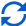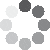Enter parameters Wall
Wall
Width:
ft
• inches (in)
• feet (ft)
• yards (yd)
• centimeters (cm)
• meters (m)
Height:
ft
• inches (in)
• feet (ft)
• yards (yd)
• centimeters (cm)
• meters (m)
No. of Doors:
No. of Windows:
Price Per:
ft²
• squares (100 ft²)
• square inches (in²)
• square feet (ft²)
• square yards (yd²)
• acres (ac)
• square meters ()
Waste (%):Result
Siding needed:
0
ft²
• square inches (in²)
• square feet (ft²)
• square yards (yd²)
• square miles (mi²)
• acres (ac)
• square centimeters (cm²)
• square meters ()
Squares:
0
Total price:
0
Gable
Width:
ft
• inches (in)
• feet (ft)
• yards (yd)
• centimeters (cm)
• meters (m)
Height:
ft
• inches (in)
• feet (ft)
• yards (yd)
• centimeters (cm)
• meters (m)
No. of Doors:
No. of Windows:
Price Per:
ft²
• squares (100 ft²)
• square inches (in²)
• square feet (ft²)
• square yards (yd²)
• acres (ac)
• square meters ()
Waste (%):Result
Siding needed:
0
ft²
• square inches (in²)
• square feet (ft²)
• square yards (yd²)
• square miles (mi²)
• acres (ac)
• square centimeters (cm²)
• square meters ()
Squares:
0
Total price:
0
Embed(No Ratings Yet)Loading...
Real-time graphics. Make the calculations and see the changes.
Real-time graphics. Make the calculations and see the changes.
Embed
Embed
Embed this calculator on your site!Add live graphics
Copied to clipboard! PreviewWhat's wrong?
Minimum 5 characters!